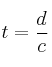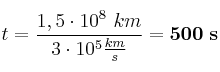# Velocity of light 0001

, por

There are 150 000 000 km between the Sun and the Earth. If the velocity of light in vacuum is 300 000 km/s, how long light takes to reach the Earth?

## SOLUCIÓN:

Clearing "time" in the velocity equation:("c" is the speed of light).
Now is time to solve the equation: